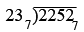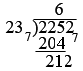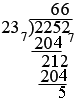SEARCH HOMEMath Central Quandaries & QueriesQuestion from Promise, a student: Simplify the following 302 in base 6 divided by 5 in base 6Promise,

I am going to illustrate the procedure by dividing 2252 by 23 in base 7

Before I actually start the division I am going to calculate 1× 237, 2 × 237, 3× 237 4× 237 5× 237 and
6× 237 by repeated addition.

1 × 237 = 237
2 × 237 = 237 + 237 = 467
3 × 237 = 467 + 237 = 1027

and so on. My resulting table is

1 × 237 = 237
2 × 237 = 46 7
3 × 237 = 102 7
4 × 237 = 125 7
5 × 237 = 151 7
6 × 237 = 204 7

Now I can start the division.I can see from my multiplication that 237 divides 2257 6 times. Thus I get, after subtractionAgain using of the table I see that 237 divides 2127 6 times and thusHence 22527 divided by 237 is 667 with a remainder of 57 .

PennyMath Central is supported by the University of Regina and The Pacific Institute for the Mathematical Sciences.